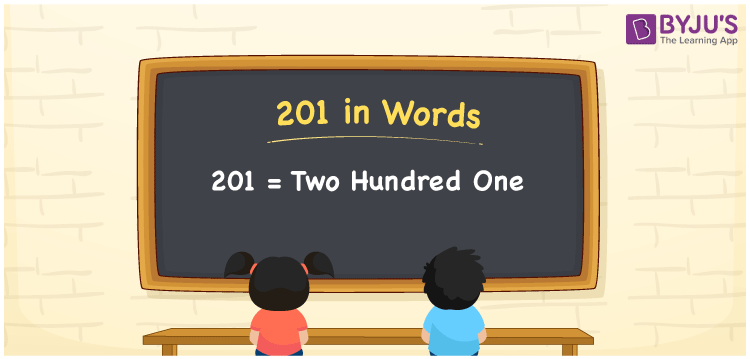# 201 in Words

201 in words can be written as Two Hundred One. If you buy a packet of biscuits for Rs. 201, then you can say that “I bought a packet of biscuits for Two Hundred One Rupees”. In this article, you will learn how numbers in words concepts can be written with the help of the English alphabet. Therefore, 201 can be read as “Two Hundred One” in words.

 201 in words Two Hundred One Two Hundred One in Numbers 201

## 201 in English Words## How to Write 201 in Words?

Learn how to write the place value chart of 201 from the in depth explanation provided below to help students grasp the basic concepts.

 Hundreds Tens Ones 2 0 1

201 in expanded form is:

2 × Hundred + 0 × Ten + 1 × One

= 2 x 100 + 1

= 200 + 1

= 201

= Two Hundred One

Therefore, 201 in words is written as Two Hundred One.

201 is a natural number that precedes 202 and succeeds 200.

201 in words – Two Hundred One

Is 201 an odd number? – Yes

Is 201 an even number? – No

Is 201 a perfect square number? – No

Is 201 a perfect cube number? – No

Is 201 a prime number? – No

Is 201 a composite number? – Yes

## Frequently Asked Questions on 201 in Words

Q1

### Write 201 in words.

201 can be written in words as “Two Hundred One”.
Q2

### How do you write Two Hundred One in numbers?

Two Hundred One can be written in numbers as 201.
Q3

### Is 201 an even number?

No, 201 is not an even number as it is not completely divisible by 2.